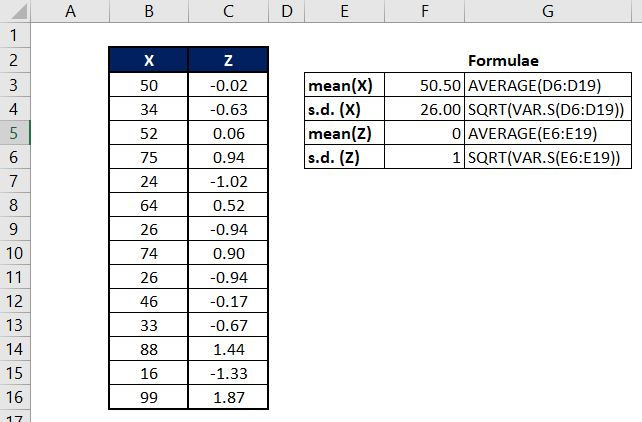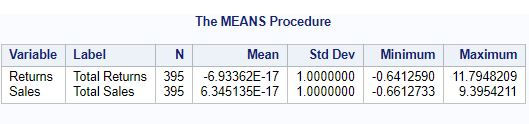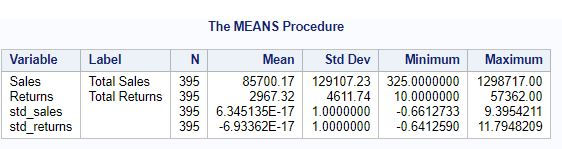top of page
Search
• Ekta Aggarwal

# PROC STANDARD - To standardize or normalize the variables.

## What is standardization and why is it needed?

Standardizing the variables becomes extremely essential in the cases when all the variables are of different scales. For eg. area of a home (in yards) and prices (in dollars) are completely different scales due to which the coefficients or the results of some data science algorithms cannot be reliable (eg. gradient descent). In order to make them comparable we need to make them on the same scale i.e. standardize them.

## How to standardize?

The most common approach of standardizing the variables is subtracting the mean of the series from each observation and then dividing the result with the standard deviation (s.d.) of the series. This is called normalizing the variables.

Statistically,To understand more: let us take a series X and we have calculated its mean and standard deviation (s.d.) as 50.5 and 26 respectively. Now we take first element of X i.e. 50 and calculate Z = (50 - mean)/ s.d. i.e. (50-50.5)/26 = -0.02 . Similarly we do it for all the observations.

Now the mean of Z is 0 and standard deviation is 1.Now let us understand how can we standardize variables in SAS.

Let us firstly define our LIBNAME as:

`libname mylib '/home/u50132927/My_datasets';`

### Syntax:

```PROC STANDARD DATA = data_to_be_standardized OUT = data_to_save_output MEAN = X STD = Y VARDEF = divisor;
VAR list_of_numeric_variables_to_standardize;
RUN;```

DATA: Data which needs to be standardized or normalized.

OUT: Location and name of output dataset.

MEAN: What should be the mean of standardized values. Default value is 0.

STD : What should be the standard deviation of standardized values. Default value is 1.

VAR: List of column names which need to be standardized. Note: Only numeric columns can be standardized.

VARDEF: divisor to calculate the standard deviation. Can take values N-1, N , WDF and WGT. We shall explain VARDEF in detail later in this tutorial.

## Learning with examples!

For this tutorial we shall be leveraging SAS' inbuilt dataset SASHELP.SHOES. To view the decimals we have set comma and decimal formats for Sales and Returns variables.

```DATA MYLIB.SHOES;
SET SASHELP.SHOES;
FORMAT Sales comma10.2 Returns comma10.2;
RUN;```

Task: Standardize Returns and Sales column in SHOES data with mean 0 and s.d. 1.

```PROC STANDARD DATA=MYLIB.SHOES MEAN=0 STD=1 OUT=MYLIB.Standardized;
VAR Returns Sales ;
RUN;```

Our data looks as follows: Note that original variables have been replaced by standardized values.Let us get the mean of these variables after standardizing.

```PROC MEANS DATA=MYLIB.Standardized;
VAR Returns Sales;
RUN;```

For both the variables mean and standard deviation are 0 and 1 respectively.### What is you need to keep the original variables and keep standardized values in a different set of variables?

SAS always replaces the original column by standardized values. To tackle this, we have to create new variables = original variables ourselves.

We have created copy of columns Returns and Sales as std_returns and std_sales.

```DATA MYLIB.SHOES;
SET SASHELP.SHOES;
FORMAT Sales comma10.2 Returns comma10.2 std_returns comma10.2 std_sales comma10.2;
std_returns = Returns;
std_sales = Sales;
RUN;```

Now we will pass std_returns and std_sales for standardization.

```PROC STANDARD DATA=MYLIB.SHOES MEAN=0 STD=1 OUT=MYLIB.STANDARDIZED;
VAR std_returns std_sales ;
RUN;```

Let us calculate the mean and standard deviation of original and standardized variables.

```PROC MEANS DATA=MYLIB.STANDARDIZED;
VAR Sales Returns std_sales std_returns;
RUN;```## Understanding VARDEF

VARDEF is the denominator for calculation of variances.VARDEF can take following values:Source: SAS documentation

By default VARDEF = DF (i.e. n-1) , where N is the number of entries in the column.

For large data, keeping the divider N or N-1 while calculating the variance won't drastically change the results.

Let us recreate our data.

```DATA MYLIB.SHOES;
SET SASHELP.SHOES;
FORMAT Sales comma10.2 Returns comma10.2 std_returns comma10.2 std_sales comma10.2;
std_returns = Returns;
std_sales = Sales;
RUN;```

Let us standardize it using VARDEF = N:

```PROC STANDARD DATA=MYLIB.SHOES MEAN=0 STD=1 OUT=MYLIB.STANDARDIZED REPLACE VARDEF = N;
VAR std_returns std_sales ;
RUN;```
```PROC MEANS DATA=MYLIB.STANDARDIZED;
VAR Sales Returns std_sales std_returns;
RUN;```Changing the value of VARDEF has not much changed the overall mean and s.d.

### WEIGHTED Standard deviation and mean in VARDEF.

Sometimes we need to allocate weights to the observation (if some observations are more important than others then observations of higher importance are assigned a higher weight) In this way their standard deviation and means are also weighted.

To understand better, let us create our data:

```DATA MYLIB.SHOES;
SET SASHELP.SHOES;
FORMAT Sales comma10.2 Returns comma10.2 std_returns comma10.2 std_sales comma10.2;
std_returns = Returns;
std_sales = Sales;
RUN;```

In the following code we are weighting our data by STORES (note the WEIGHT statement) and we have defined VARDEF = WGT. Thus VARDEF will calculate weighted mean and weighted standard deviation of the variables and then standardize it.

```PROC STANDARD DATA=MYLIB.SHOES MEAN=0 STD=1 OUT=MYLIB.STANDARDIZED VARDEF  = WGT;
WEIGHT Stores;
VAR std_returns std_sales ;
RUN;```

Let us calculate the means and standard deviation:

```PROC MEANS DATA=MYLIB.STANDARDIZED;
VAR Sales Returns std_sales std_returns;
RUN;```Weighting the data by stores has shifted the mean and s.d.

See All
bottom of page